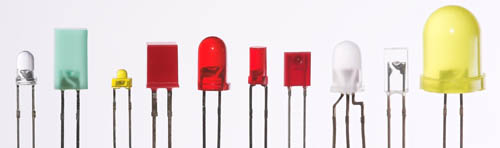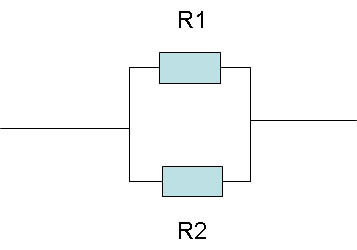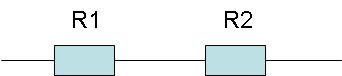# Electronics Primer: Introduction

The field of electronics offers a powerful set of tools for obtaining accurate numerical data. Instead of just saying that there is a difference between two things (color, brightness, charge, etc.), electronic devices allow you to measure precisely how big the difference is.

Electrons and Charge

The word electronics is derived from "electron." Electrons are sub-atomic particles with a negative charge. The unit for electric charge is the coulomb. One coulomb equals the charges of 6.24 billion billion (1018) electrons. A single electron has a charge that is too small to measure in most electronic devices, so scientists use coulombs as a more useful way to describe charge.

Multimeters

The basic outputs for electronic devices are voltage, current, and resistance. Inexpensive and sensitive devices, called multimeters, can measure each of these. If you can devise a way for the output of your experiment to be in the form of voltage, for example, you can use a multimeter to get precise numerical data. For more information about using a multimeter, see the Science Buddies reference How to Use a Multimeter.

Voltage

The definition of voltage is: the measurement of the potential for an electric field to cause a current in a conductor. An electric field "pushes and pulls" electric charges, so if you put an electron in an electric field, it will move. The movement of charged particles is a current (more about current below). The essential point is that the voltage is a measure of how strongly charged particles are being pushed and pulled by an electric field. The symbol for voltage is V.

Consider a simple flashlight with two D batteries. Each D battery has a voltage of 1.5 V. By putting two 1.5-V batteries together, the total voltage equals 3 V. This voltage is high enough to power a lightbulb. When you turn the flashlight on, the voltage difference causes electrons to flow through the lightbulb, making it shine. The electric field provides the energy to move charged particles through wires (electrical conductors) and through the lightbulb.

Voltage can be direct (DC) or alternating (AC). In DC voltage, the voltage does not alternate. If you graph the voltage of a 9-V battery vs. time, for example, you will have a straight line at a value of 9 V. Alternating current flips back and forth between positive and negative. If you make a graph of AC current vs. time, it will alternate from positive to negative, often in the form of a sine wave. Voltage is supplied to a circuit by a battery or other power supply.

Current

Current is a measurement of how much charge moves through a circuit in a given period of time. In the case of the flashlight, the current through the lightbulb is a measurement of the amount of electric charge flowing through the lightbulb in a given time.

The symbol for current is I. The symbol for the unit of current, the ampere, is A. The precise definition of an ampere is: the current produced by the flow of one coulomb per second. Use "I" when referring to current (as in Ohm's law, discussed below) and "A" when referring to the amount of current.

DC current is produced by DC voltage and AC current is produced by AC voltage.

Resistance

Electrons flow through materials in response to a voltage, creating a current. Some materials, such as copper, have very low resistance, so the electrons flow freely—they are good conductors. Some materials have intermediate resistance, such as the semiconductors used to make transistors. Semiconductors might have a threshold value for the voltage that will cause a current to flow, for example. And some materials, such as rubber, have high resistance and are used as insulators to separate charges.

The symbol for resistance is R. The unit for resistance is the ohm, which has the symbol Ω, and is the capital letter "W" in Greek.

Ohm's Law

Ohm's law relates voltage, current, and resistance, mathematically. Ohm's law can be written as: $V = IR$ . In words, Ohm's law states that the voltage in a component in a circuit equals the current through the component, times the resistance of the component.

Electronic Circuit

An electronic circuit is a closed path formed by the interconnection of electronic components through which an electric current can flow. You might find it helpful to compare an electronic circuit to a circuit in which water flows.

• Voltage in the electronic circuit is like the pump in the water circuit—it provides the push to make things go.
• Current in the electronic circuit is like the rate (in liters per second, for example) that water flows in the water circle.
• And resistance in an electronic circuit is like a constriction in a hose in the water circuit. An electronic resistor impedes the flow of electrons, just as a constriction in a hose impedes the flow of water.
• AC - Abbreviation for alternating current, which is voltage that flips back and forth between positive and negative.
• Ampere - Unit of current (symbol: A).
• Breadboard - A board used to make temporary circuits. The breadboard has metal-lined sockets for connecting electronic components in a test circuit.
• Capacitor - An electronic component consisting of two conducting surfaces, separated by an insulator. It is used to store and release energy and to control high-frequency signals.
• Circuit - A collection of electronic parts connected together, usually designed to perform some kind of function.
• Circuit diagram - A diagram that depicts a circuit, using symbols for electronic components. Used to design and communicate circuits with other people, like a blueprint or a plan.
• Closed circuit - A circuit in which current can flow through electronic components, from a point of high voltage to a point of low voltage.
• Conductance - The opposite of resistance. Materials with high conductance (e.g. metals) have low resistance. The unit of conductance is siemens (S).
• Current - The flow of electric charge. The unit for current is amperes (A).
• DC - Abbreviation for direct current voltage, which is voltage that does not alternate.
• Diode - An electronic component that allows current to flow freely in only one direction.
• I - Symbol for current. The unit for current is the ampere (A).
• Integrated circuit (IC) - An electronic component that contains several simpler electronic components. An IC is a miniaturized electronic circuit.
• Jumper - A short length of wire used to temporarily complete a circuit or to bypass a break in a circuit.
• Kilo - A prefix meaning "thousand." A 10-kΩ resistor is 10,000 ohms.
• Lead - Length of wire used to make connections between components in a circuit.
• Light-emitting diode (LED) - A solid-state device that has two key features: it allows current to flow in only one direction (that is the "diode" part), and it emits light when current flows through it in the "allowed" direction. LEDs are described by several specifications, some of the more important of which are:
• Maximum current;
• Brightness;
• Color of light;
• Angle of light beam (for example, an LED with an angle of 15 degrees produces a more focused beam than one with a beam of 45 degrees); and
• Size, usually 5 mm.Figure 1. Light-emitting diodes come in a variety of sizes, shapes, and colors. (Wikipedia, 2008.)

• Mega - A prefix meaning "million" (symbol: M). A 1-MΩ (megaohm) resistor has a resistance of 1 million ohms.
• Multimeter - An instrument with several different kinds of meters. Multimeters are used to measure voltage, current, and resistance, among other things.
• Ohm - Unit of resistance. The symbol for the ohm is Ω. You can make this symbol in Microsoft Word by changing the font of a capital "W" into the font called "Symbol," using the "Format" menu.
• Ohm's law - V = IR, or "Voltage equals current times resistance." Example: Say a 1,000-Ω resistor has a voltage drop of 9 V. What is the amount of current that is flowing through the resistor? Plugging the numbers in yields 9 V = I amps x 1,000 Ω, so I = 9/1,000 amps (A), or 9 milliamps (mA).
• Open circuit - A circuit with a gap in it that prevents current from flowing. When you turn of a light with a light switch, you open up the circuit connecting the lightbulb to the source of electricity.
• Parallel connection - Current flows through two or more components at the same time. As an analogy, imagine a river splitting into two or more parallel streams, which then merge together again. The amount of current flowing through the various "streams" depends on their resistance.Figure 2. This diagram shows two resistors in a parallel connection.

• R - Symbol for resistance. The electrical resistance of an object is a measure of its opposition to the passage of a steady electrical current. Metals have very low resistance, whereas substances such as rubber and plastic have high resistance. The unit of resistance is the ohm, with the symbol Ω.
• Resistor - An electronic component used to "resist" the flow of current. Resistors are used to control current in an electric circuit. The unit of resistance is the ohm (Ω). The value for the resistance of a resistor is coded by colored lines on the resistor. When they are in a circuit with a voltage supply, a "voltage drop" occurs across the resistor.

Resistors have three key features: their level of resistance, their power rating, and their tolerance. Say a resistor has a resistance of 220 ohms (Ω), a power rating of 1/4 watt (W), and a tolerance of 5 percent. The power rating tells you the limit of the power (power = current x voltage through the resistor) that the resistor can withstand without overheating. Tolerance is a measure of the resistance range. A 220-ohm resistor with a 5 percent tolerance will have a resistance in the range of 220 ohms ± 11 ohms. An electrical specification might call for a resistor with a value of 100 Ω (ohms), but will also state a tolerance, such as "±1%". This means that any resistor with a value in the range 99 Ω–101 Ω is acceptable.

• Short circuit - A low-resistance connection established between two points in an electric circuit that are designed to be at different voltages. For example, connecting the positive and negative poles of a battery with a wire causes a short circuit. Because of the low resistance of the connection, the level of current can be very high, leading to excessive heat and damage to the circuit. Short circuits can cause fires and explosions if the current is large enough.
• Serial connections - In a serial connection, the current flows through each component one at a time, like a river passing through several dams. The amount of current flowing through the components is equal, since they are connected "end-to-end." Compare to parallel connection.Figure 3. In this diagram, two resistors are shown in a serial connection.

• Tolerance - The range of variation permitted in an electrical component.
• V - Symbol for voltage. The unit for voltage is the volt.
• Voltage - A measure of the difference in electric potential between two points. A voltage difference causes electrons to flow, much like a difference in height can make water flow. The unit for voltage is the volt (V).
• Winscope - A free program that lets you use your computer as an oscilloscope. It is very useful for measuring voltage vs. time data for beginner electronics projects.